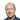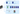# Factorization of MSI data - part 1

##### This post is part of our series titled "Unsupervised learning on MSI data", which contains the following entries:Factorization of MSI data - part 1 (current post)Non-negative matrix factorization in MSI

• Introduction
• Matrix factorization in a nutshell
• Principal Component Analysis (PCA)
• Varimax
• Independent Component Analysis (ICA)
• Conclusion

• ## Introduction

##### Unsupervised data analysis is primarily targeted at exploring the content of the data, and extracting the trends present in a mostly unbiased way. In contrast to supervised methods, these methods do not require any labelling or prior information on the data. When such information is available, (semi-)supervised methods are generally the recommended way to go. If you are not yet familiar with MSI technology and its associated data, please refer to our introductory post on MSI data analysis.

Introduction to MSI data analysis

We recently published a review paper on unsupervised analysis of MSI data together with profs. Raf Van de Plas (TU Delft) and Richard Caprioli (Vanderbilt University). For in-depth information about the topics we touch upon in this blog series, please consult our review paper and its references.
##### A wide array of techniques has been used in the unsupervised analysis of MSI data, which can broadly be broken down into 3 main categories, namely factorization methods, clustering methods and manifold learning or non-linear dimensionality reduction techniques, as shown in Figure 1.## Matrix factorization in a nutshell

##### Matrix factorization techniques are an important class of methods used in unsupervised MSI data analysis. Matrix factorization methods take a large and often high-dimensional dataset acquired by a MSI experiment (or several experiments), and decompose it into a (typically reduced) number of trends that underlie the observed data.
These approaches are applied to the matrix representation commonly used for MSI data sets, as discussed in our introductory post on MSI data analysis. When representing MSI data in matrix form, D is a data matrix where rows denote pixels and columns denote m/z bins. Hence, for a single MSI data set, m is the number of pixels and n is the number of spectral bins or m/z bins, i.e. each row of D represents the mass spectrum of a pixel in the sample along a common m/z binning.

## Principal Component Analysis (PCA)

##### The first PC is defined such that it captures the largest possible amount of variance in the data. Each subsequent PC is uncorrelated to the previous PCs and describes the largest possible variance that remains in the data after removal of the preceding PCs. Roughly speaking, this means that the largest trend in the MSI data will be captured by the first PC, the second largest trend by the second PC, etc.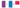### PCA applied to toy data

##### If we are interested in the large trends in the data, we can discard PC2, and still maintain a lot of that variability in our original data. This said, we will lose the “information” along PC2 if we do so. In this simple case we can see that we probably would not lose a lot of information if PC2 is discarded, as that direction contains mostly noise.
Note that, while we started with all-positive data on axes 1 and 2 initially, the origin of the new orthogonal axes defined by PCA is placed at the center of where the variance occurs (mean subtraction). This will result in negative values when we project our data onto these new axes, which will be an important point below.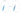### PCA on an image

##### Finally, we see that PC3 and PC4 provide additional information, but focus increasingly more on smaller parts of the image. This also shows that the number of components that are relevant depend on the the goal of the analysis. If the goal was to recognize the character, we would have enough with PC1 alone, and could discard the other components, but we’ll come back to that later.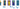### PCA applied to MSI data

##### When applying PCA to MSI data, the resulting score matrix extracts trends in the pixel space, i.e. spatial trends (the singular vectors spanning the pixel space), whereas the loading matrix extracts spectral trends (singular vectors spanning the spectral space) from the data. Figure 5 shows the 10 first principal components, with the spatial expression on the left, and the matching spectral expression on the right.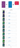### Interpretation

##### As a general rule, peaks that have a high absolute intensity in the pseudo-spectrum will have an important role in the spatial expression of the region highlighted by the principal component.
Some caveats must be made: ideally, this pseudo-spectrum would give a straightforward list of which bio-moleculecular ions are involved in each region from a biological sense. However, as we said before, the goal of PCA is to capture and summarize as much of the variation in the data as possible per principal component. This is not necessarily the same as correctly modeling the underlying biology or sample content. It’s a bit like you had only one sheet of paper to summarize an entire book and went a bit overboard. While it may capture the essence of the book, the end result might not be too reader-friendly or clear on what everything means.

### Selecting the number of principal components

##### An important issue when using PCA is the number of components to retain. This is not just a problem for PCA, but for nearly any dimensionality reduction method, be it factorization or clustering. When using factorization in the context of MSI, we hope to get a much more concise representation of the original data by grouping together m/z bins that operate together, such that we end up with tens to hundreds of components compared to the original thousands to millions of variables. In PCA, each subsequent principal component will capture less and less of the variation in the data until we end up with components that just capture noise. Identifying that cut-off point is difficult and there is no clear cut answer to this problem, however, an often-used method is the cumulative variance plot, which shows the percentage of variance that each additional component explains. This can be used to set a cut off point for how many components to retain, based on the amount of variance that is captured.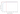## Varimax

##### PCA, as well as many other factorization methods, suffer from what is known as ‘rotational ambiguity’. Above we showed that the result of a PCA analysis is a new set of orthogonal axes onto which the data is projected. We can rotate these axes and still represent the same amount of information, i.e. there are an infinite number of ways in which we can orient the axes without losing any “information”, as is illustrated in Figure 7.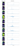##### Using PCA+Varimax involves the following steps:
1. Compute the PCA composition with a certain (relatively large) number of components, typically 100-500 though this depends on the dataset and use-case.
2. Determine the number of PCs that must be retained () to capture sufficient variation within the data, for instance using a Pareto chart as in Figure 5.
3. Compute the Varimax rotation on the first PCs to obtain the new basis (which spans the same subspace as the original PCs).
##### Note that steps 1 and 2 are identical to PCA, only the rotation in step 3 is new.
There are two important caveats with using Varimax. First off, unlike PCA, the components are no longer ranked by variance i.e. “importance”, so we would have to look at all 50 components to get a good image of what is going on. Nonetheless, when applying a Varimax rotation to N principal components, the exact same amount of variance is explained as in PCA with N components. Secondly, unlike PCA, Varimax does not have an analytical solution; it is an iterative algorithm, and a global optimum is not guaranteed. This means that running the algorithm multiple times will generally result in different solutions of comparable quality.

## Independent Component Analysis (ICA)

##### As said, ICA originates in blind source separation theory, where a well known example to illustrate the goal at hand is the cocktail party problem. This example, featured in Figure 9, has the premise that a party is held in a room, and microphones are placed around different locations in that room. The microphones all pick up the same party, but microphone 1 is located closer to group of guests 1 than microphone 2. The aim of the algorithm is to find the different “sources” (here e.g. the speakers) from which sound in the party is originating, and reconstruct their original signals (here e.g. the conversations).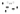##### An additional motivation for using ICA over PCA is that one of the underlying assumptions of PCA is that the underlying variables have a normal distribution, which is generally not true for mass spectrometry imaging data. The intensities of a peak in a mass spectrum are the result of a counting process, and are thus Poisson distributed, rather than normally distributed. Furthermore, biological processes often give rise to non-normally distributed signals (e.g. feedback loops). In fact, most real-life data is not normally distributed, and ICA instead uses such non-normal features of the data to find the underlying signals. ICA algorithms will often use PCA as a preprocessing step to perform dimensionality reduction, and to “whiten” the data, i.e. remove any correlations from it.
Caveats: Similar to Varimax, fastICA does not rank the components and does not have an analytical solution. Likewise a global optimum is not guaranteed and running the algorithm multiple times will generally result in different solutions.
##### Below we’ve applied the FastICA algorithm available in scikit-learn to our dataset, setting the number of components to 50 based on our variance plot, and the number of iteration per component to 1000. Compared to PCA, the spatial expressions seem to be more focused to specific regions in the tissue. In our results, we saw some spatial structures that did not immediately show up in the PCA and PCA+Varimax decompositions. Something that we’ve observed in the past is that often the ICA pseudo-spectra contain less of a mixture of negative and positive peaks, although this is not very obvious in our current example. While it is difficult to say which decomposition is the better one, without a ground truth, in my PhD thesis (which you can find here). I did some experiments with artificially constructed MSI datasets, where ICA was better at retrieving the original artificial spectra than PCA.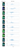## Conclusion

##### This post is part of our series titled "Unsupervised learning on MSI data", which contains the following entries:Factorization of MSI data - part 1 (current post)Non-negative matrix factorization in MSI